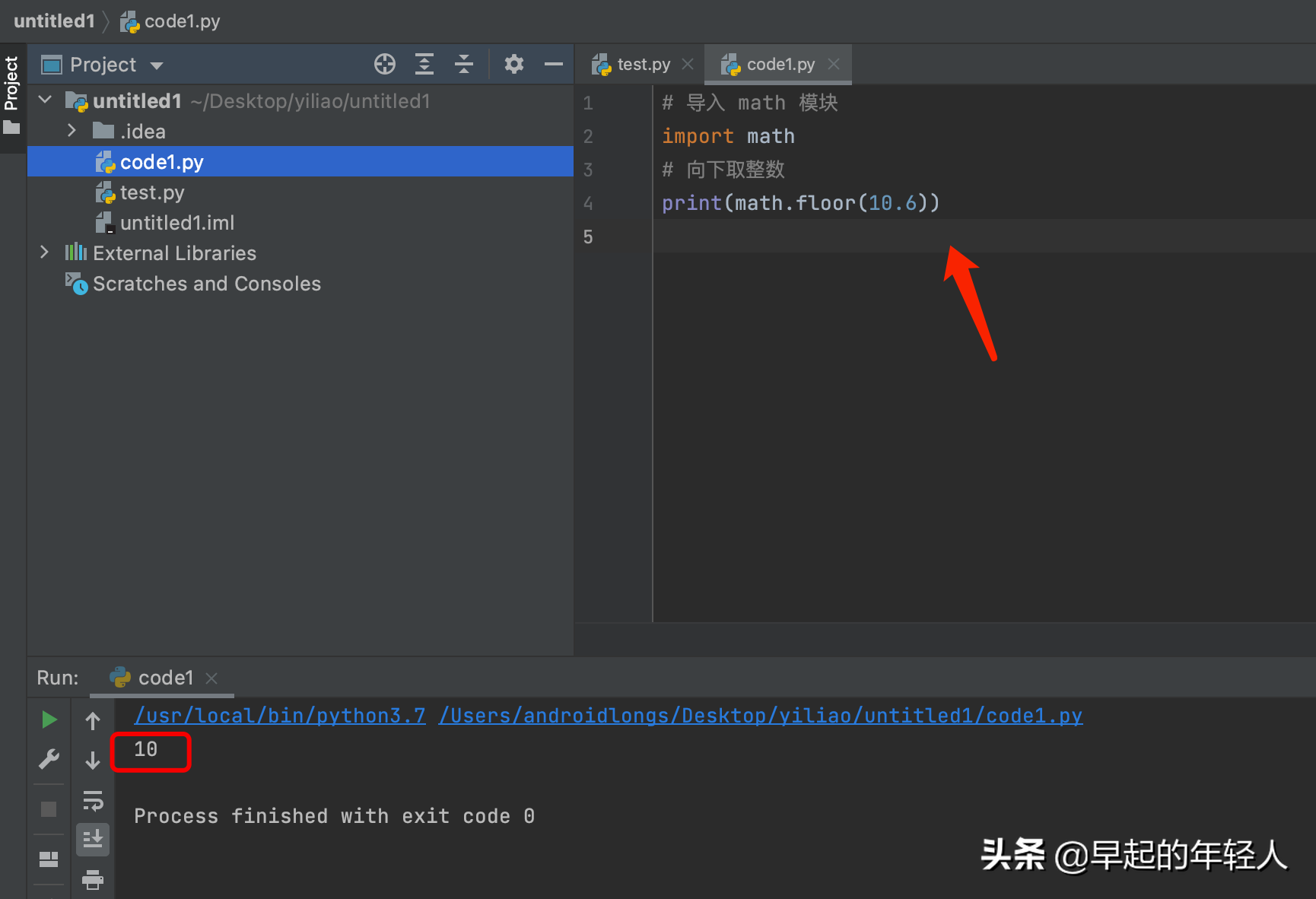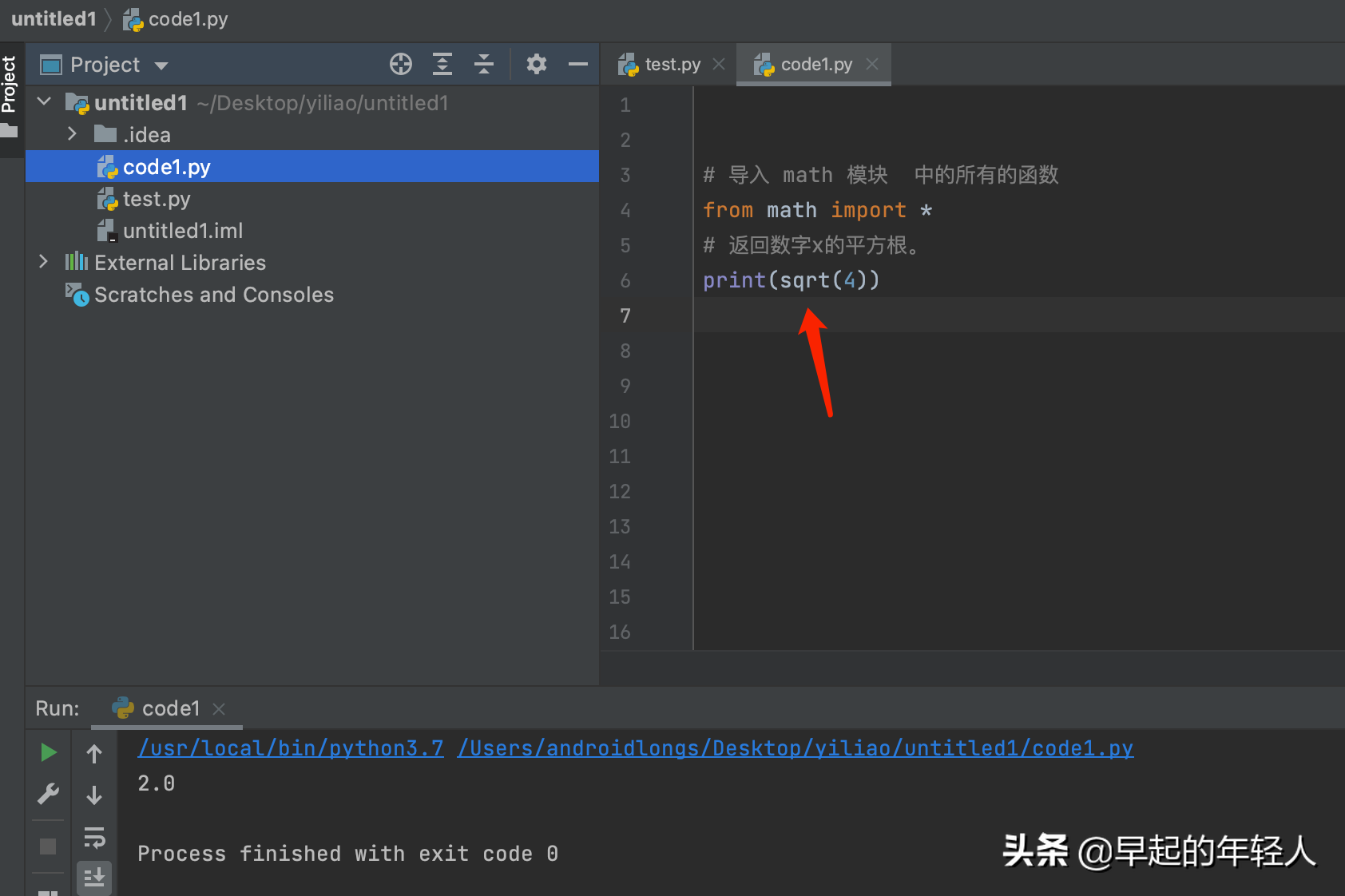• A+

1 Python的语言基础 导入模块

import module_name

module_name.function.name

from module_name import function_name

from module_name import *

2 实例应用

math 模块是 python中用于数字计算的相关模块

2.1 导入整个math 模块

# 导入 math 模块
import math
# 向下取整数
print(math.floor(10.6))2.2 导入math 模块中的 sin 函数方法

# 导入 math 模块 sin 函数方法
from math import sin
# 正弦值
print(sin(10.6))2.3 导入math 中的所有的函数

# 导入 math 模块  中的所有的函数
from math import *
# 返回数字x的平方根。
print(sqrt(4))